# Regularize Logistic Regression

This example shows how to regularize binomial regression. The default (canonical) link function for binomial regression is the logistic function.

### Step 1. Prepare the data.

Load the `ionosphere` data. The response `Y` is a cell array of `'g'` or `'b'` characters. Convert the cells to logical values, with `true` representing `'g'`. Remove the first two columns of `X` because they have some awkward statistical properties, which are beyond the scope of this discussion.

```load ionosphere Ybool = strcmp(Y,'g'); X = X(:,3:end); ```

### Step 2. Create a cross-validated fit.

Construct a regularized binomial regression using 25 `Lambda` values and 10-fold cross validation. This process can take a few minutes.

```rng('default') % for reproducibility [B,FitInfo] = lassoglm(X,Ybool,'binomial',... 'NumLambda',25,'CV',10); ```

### Step 3. Examine plots to find appropriate regularization.

`lassoPlot` can give both a standard trace plot and a cross-validated deviance plot. Examine both plots.

```lassoPlot(B,FitInfo,'PlotType','CV'); legend('show','Location','best') % show legend ```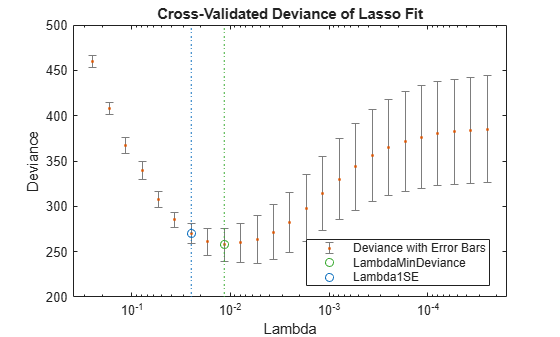The plot identifies the minimum-deviance point with a green circle and dashed line as a function of the regularization parameter `Lambda`. The blue circled point has minimum deviance plus no more than one standard deviation.

```lassoPlot(B,FitInfo,'PlotType','Lambda','XScale','log'); ```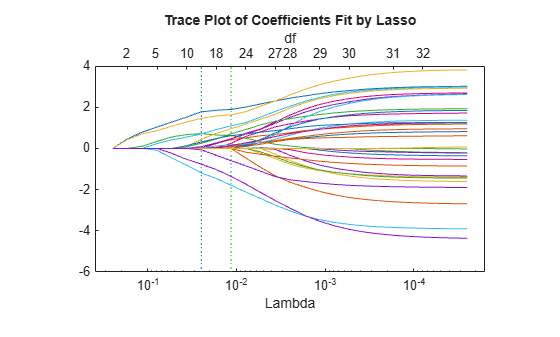The trace plot shows nonzero model coefficients as a function of the regularization parameter `Lambda`. Because there are 32 predictors and a linear model, there are 32 curves. As `Lambda` increases to the left, `lassoglm` sets various coefficients to zero, removing them from the model.

The trace plot is somewhat compressed. Zoom in to see more detail.

```xlim([.01 .1]) ylim([-3 3]) ```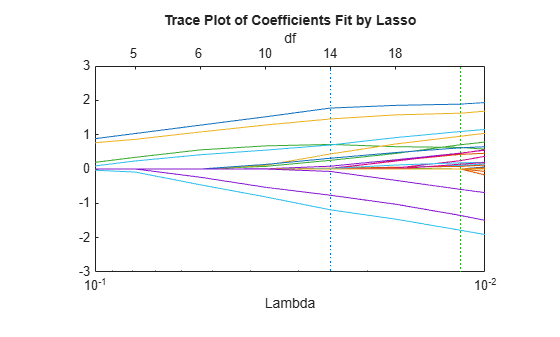As `Lambda` increases toward the left side of the plot, fewer nonzero coefficients remain.

Find the number of nonzero model coefficients at the `Lambda` value with minimum deviance plus one standard deviation point. The regularized model coefficients are in column `FitInfo.Index1SE` of the `B` matrix.

```indx = FitInfo.Index1SE; B0 = B(:,indx); nonzeros = sum(B0 ~= 0) ```
```nonzeros = 14 ```

When you set `Lambda` to `FitInfo.Index1SE`, `lassoglm` removes over half of the 32 original predictors.

### Step 4. Create a regularized model.

The constant term is in the `FitInfo.Index1SE` entry of the `FitInfo.Intercept` vector. Call that value `cnst`.

The model is logit(mu) = log(mu/(1 - mu)) = `X*B0 + cnst` . Therefore, for predictions, mu = `exp(X*B0 + cnst)/(1+exp(x*B0 + cnst))`.

The `glmval` function evaluates model predictions. It assumes that the first model coefficient relates to the constant term. Therefore, create a coefficient vector with the constant term first.

```cnst = FitInfo.Intercept(indx); B1 = [cnst;B0]; ```

### Step 5. Examine residuals.

Plot the training data against the model predictions for the regularized `lassoglm` model.

```preds = glmval(B1,X,'logit'); histogram(Ybool - preds) % plot residuals title('Residuals from lassoglm model') ```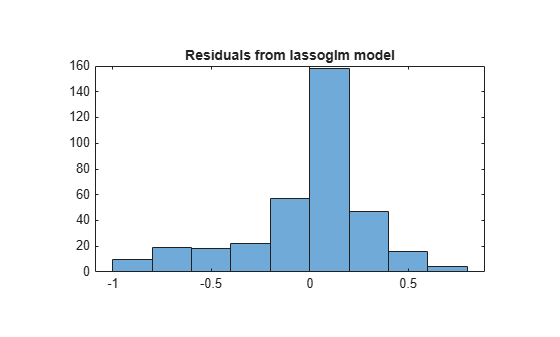### Step 6. Alternative: Use identified predictors in a least-squares generalized linear model.

Instead of using the biased predictions from the model, you can make an unbiased model using just the identified predictors.

```predictors = find(B0); % indices of nonzero predictors mdl = fitglm(X,Ybool,'linear',... 'Distribution','binomial','PredictorVars',predictors) ```
```mdl = Generalized linear regression model: y ~ [Linear formula with 15 terms in 14 predictors] Distribution = Binomial Estimated Coefficients: Estimate SE tStat pValue _________ _______ ________ __________ (Intercept) -2.9367 0.50926 -5.7666 8.0893e-09 x1 2.492 0.60795 4.099 4.1502e-05 x3 2.5501 0.63304 4.0284 5.616e-05 x4 0.48816 0.50336 0.9698 0.33215 x5 0.6158 0.62192 0.99015 0.3221 x6 2.294 0.5421 4.2317 2.3198e-05 x7 0.77842 0.57765 1.3476 0.1778 x12 1.7808 0.54316 3.2786 0.0010432 x16 -0.070993 0.50515 -0.14054 0.88823 x20 -2.7767 0.55131 -5.0365 4.7402e-07 x24 2.0212 0.57639 3.5067 0.00045372 x25 -2.3796 0.58274 -4.0835 4.4363e-05 x27 0.79564 0.55904 1.4232 0.15467 x29 1.2689 0.55468 2.2876 0.022162 x32 -1.5681 0.54336 -2.8859 0.0039035 351 observations, 336 error degrees of freedom Dispersion: 1 Chi^2-statistic vs. constant model: 262, p-value = 1e-47 ```

Plot the residuals of the model.

```plotResiduals(mdl) ```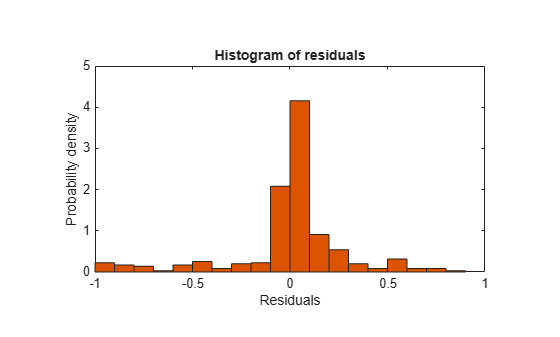As expected, residuals from the least-squares model are slightly smaller than those of the regularized model. However, this does not mean that `mdl` is a better predictor for new data.